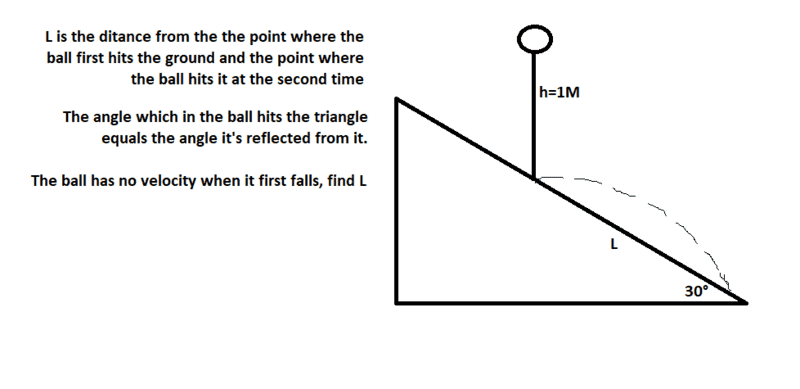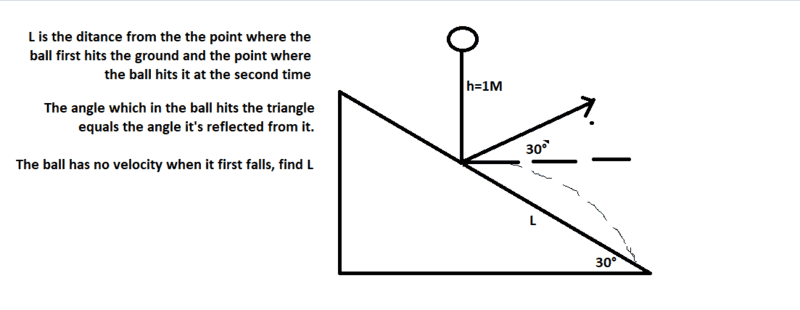# Can this problem be solved without energy considerations?

## Homework Statement## The Attempt at a Solution

I wrote equations for the vertical and horizonal distances, but I still wasn't able to find L (was missing an equation).
Is it possible?

PeroK
Homework Helper
Gold Member
2020 Award

## Homework Statement

View attachment 216959

## The Attempt at a Solution

I wrote equations for the vertical and horizonal distances, but I still wasn't able to find L (was missing an equation).
Is it possible?

You need to show us how far you got.

As I see it, given the information, the ball can only land in one position, so it must be possible to solve the problem.

PS I think you are supposed to assume energy conservation from the angle of incidence being equal to angle of reflection.

gneill
Mentor
You'll need to provide the details of your work.

It's certainly possible to use kinematic equations to find the result. The acceleration due to gravity is a constant value, hence the constant acceleration kinematic formulae all apply (SUVAT).

Edit: Ah! @PeroK got there ahead of me!

You need to show us how far you got.

As I see it, given the information, the ball can only land in one position, so it must be possible to solve the problem.

PS I think you are supposed to assume energy conservation from the angle of incidence being equal to angle of reflection.
You'll need to provide the details of your work.

It's certainly possible to use kinematic equations to find the result. The acceleration due to gravity is a constant value, hence the constant acceleration kinematic formulae all apply (SUVAT).

Edit: Ah! @PeroK got there ahead of me!

First we can know this:Now lets say that the ball leaves the surface with a velocity equals to V.
We can say that:
Lcos(30)=Vcos(30)t
-Lsin(30)=Vsin(30)t-5t2.
We see at the end that V=5t, and that L=0.2V2.
We also know that the ball hits the surface with a velocity of √(20), all vertical.
Now I am stuck.

#### Attachments

You need to show us how far you got.

As I see it, given the information, the ball can only land in one position, so it must be possible to solve the problem.

PS I think you are supposed to assume energy conservation from the angle of incidence being equal to angle of reflection.
We solved this problem in class before we studied energy, so my teacher either used it to introduce the topic to us or solved it without it.

gneill
Mentor
Now lets say that the ball leaves the surface with a velocity equals to V.
We can say that:
Lcos(30)=Vcos(30)t
-Lsin(30)=Vsin(30)t-5t2.
We see at the end that V=5t, and that L=0.2V2.
We also know that the ball hits the surface with a velocity of √(20), all vertical.
Now I am stuck.
You can use the first equation to find an expression for ##t##. You can substitute that along with the known values for sin(30), cos(30), and V into the second equation to yield an equation with only one unknown, ##L##.

You can use the first equation to find an expression for ##t##. You can substitute that along with the known values for sin(30), cos(30), and V into the second equation to yield an equation with only one unknown, ##L##.
Didn't you use energy considerations if you assumed that the velocity stays the same after the ball hits the surface?

PeroK
Homework Helper
Gold Member
2020 Award
First we can know this:
View attachment 216960
Now lets say that the ball leaves the surface with a velocity equals to V.
We can say that:
Lcos(30)=Vcos(30)t
-Lsin(30)=Vsin(30)t-5t2.
We see at the end that V=5t, and that L=0.2V2.
We also know that the ball hits the surface with a velocity of √(20), all vertical.
Now I am stuck.

I think you've almost got it. You have:

##L = 0.2 V^2##

I assume that is with ##g =10m/s^2##.

And, you have got ##V = \sqrt{20} m/s##

So, that's it isn't it? It bounces off the triangle with the same speed ##V## as it hit it. Just in a different direction.

I think you've almost got it. You have:

##L = 0.2 V^2##

I assume that is with ##g =10m/s^2##.

And, you have got ##V = \sqrt{20} m/s##

So, that's it isn't it? It bounces off the triangle with the same speed ##V## as it hit it. Just in a different direction.

What I am asking is how you know that the velocity stays the same after it hits the surface.

PeroK
Homework Helper
Gold Member
2020 Award
What I am asking is how you know that the velocity stays the same after it hits the surface.

Two reasons. 1) That's a property of angle of incidence = angle of reflection. 2) If you assume variable ##V## after the bounce, then the problem can't be solved! ##L## would depend on a thing called the coefficient of restitution.

Two reasons. 1) That's a property of angle of incidence = angle of reflection. 2) If you assume variable ##V## after the bounce, then the problem can't be solved! ##L## would depend on a thing called the coefficient of restitution.
Alright, thanks a lot.

gneill
Mentor
Didn't you use energy considerations if you assumed that the velocity stays the same after the ball hits the surface?
In a way, yes: I made the practical assumption of it being a perfectly elastic collision. There was nothing in the problem statement that would suggest otherwise. See also @PeroK 's response.

haruspex